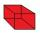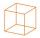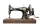# Cube

The sum of lengths of cube edges is 69 cm. What is its surface and volume?

Result

S =  198.4 cm2
V =  190.1 cm3

#### Solution:Leave us a comment of example and its solution (i.e. if it is still somewhat unclear...):

Showing 0 comments:Be the first to comment!#### To solve this verbal math problem are needed these knowledge from mathematics:

Tip: Our volume units converter will help you with converion of volume units.

## Next similar examples:

1. Edges or sidesCalculate the cube volume, if the sum of the lengths of all sides is 276 cm.
2. Cube surface areaWall of cube has content area 99 cm square. What is the surface of the cube?
3. Cube basicsHow long is the edge length of a cube with volume 23 m3?
4. Special cubeCalculate the edge of cube, if its surface and its volume is numerically equal number.
5. CubeCalculate the surface cube with edge 11 dm.
6. Cube cornersThe wooden cube with edge 64 cm was cut in 3 corners of cube with edge 4 cm. How many cubes of edge 4 cm can be even cut?
7. Volume of cubeSolve the volume of a cube with width 26cm .
8. AquariumAquarium is cube with edge 45 cm. How much water can fit in there?
9. Cube rootFor 13, Sam wrote 2891 instead of the correct cube number. By how much was he wrong?
10. Lengths of the poolMiguel swam 6 lengths of the pool. Mat swam 3 times as far as Miguel. Lionel swam 1/3 as far as Miguel. How many lengths did mat swim?
11. SewingThe lady cut off one half of cloth. She needed three-quarters of this piece to sew a skirt. What part of the original piece of cloth still remained?
12. ZdeněkZdeněk picked up 15 l of water from a 100-liter full-water barrel. Write a fraction of what part of Zdeněk's water he picked.
13. Cylindrical tank 2If a cylindrical tank with volume is used 12320cm raised to the power of 3 and base 28cm is used to store water. How many liters of water can it hold?
14. Water 31Richard takes 3 1/6 liters of water before noon and 2 3/5 liters of water after noon. How many litres of water does Richard consume a day ?
15. The shopThe shop has 3 hectoliters of water. How many liter bottles is it?
16. AreaCalculate: ?
17. Addition of Roman numbersAdded together and write as decimal number: LXVII + MLXIV